ENGR338 Digital Electronics 2021 Spring
Lab 9 - Design a Simple 8-bit ALU
Name: Ryan Jeanes

Email: rejeanes@fortlewis.edu

Designing a Simple 8-bit ALU
Introduction

Arithmetic Logic Units (ALU) are integrated circuits within a CPU or GPU that performs arithmetic and logic operations. A simple ALU is comprised of 3 2-to-1 MUXs, 4 inverters, an AND gate, an OR gate, and a Full Adder (FA). We built the schematic and layout for an 8-bit ALU and verified its logic using ElectricVLSI.

Methods and Materials

We used ElectricVLSI to design the schematic and layout of an 8-bit ALU using 3 2-to-1 MUXs, 3 inverters, an 8-bit inverter, an 8-bit AND gate, an 8-bit OR gate, and a high speed FA. We had to create an 8-bit inverter to complete the design. After creating the ALU schematic and layout we verified the logic for the AND/OR operations and addition/subtraction operations.

Results

The 8-bit ALU was built and passed DRC and NCC checks. The simulations show that the 8-bit ALU is functioning as expected.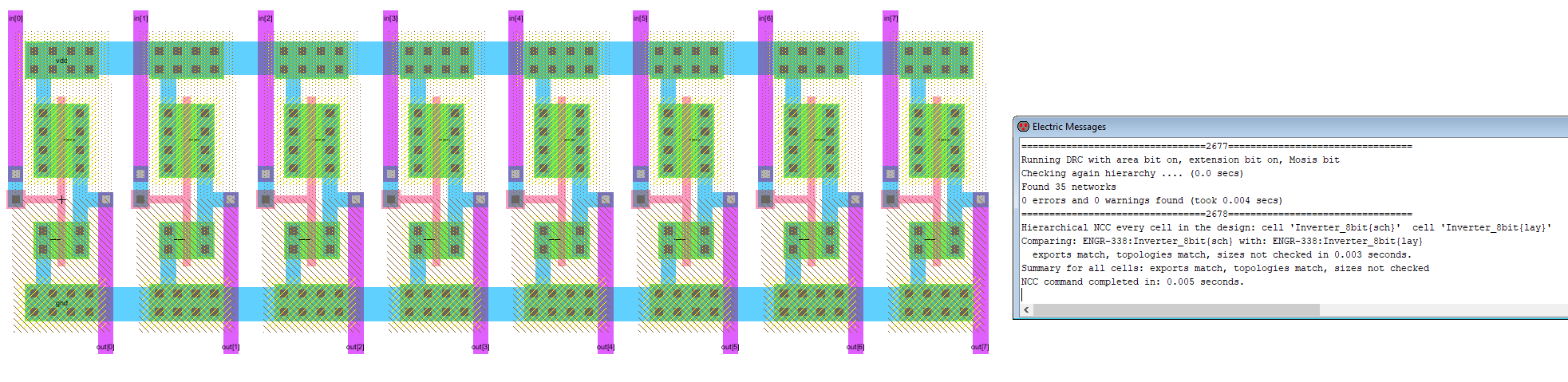Figure 1 - Layout of the 8-bit Inverter w/ DRC and NCC checks passed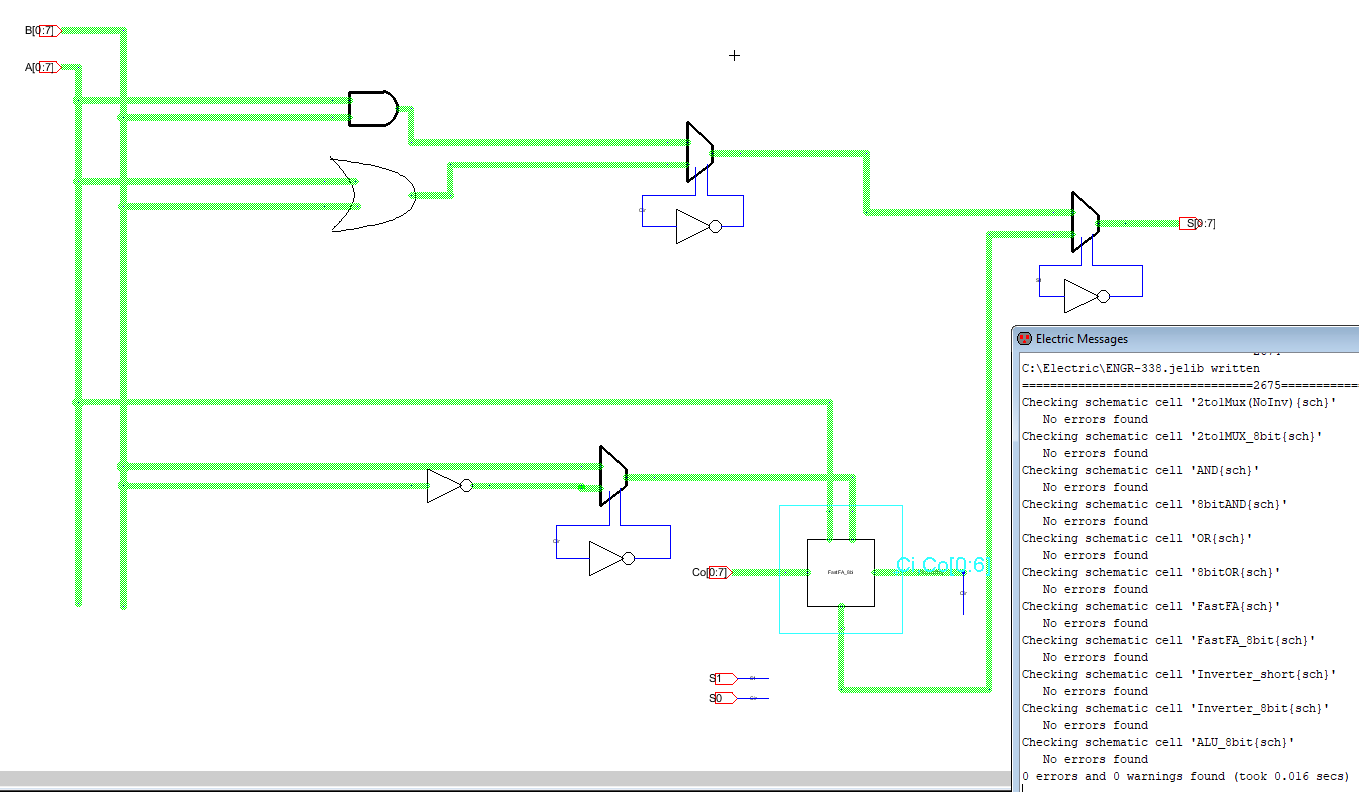Figure 2 - Schematic of the 8-bit ALU with DRC checks passed.Figure 3 - Layout of the 8-bit ALU w/ DRC and NCC checks passed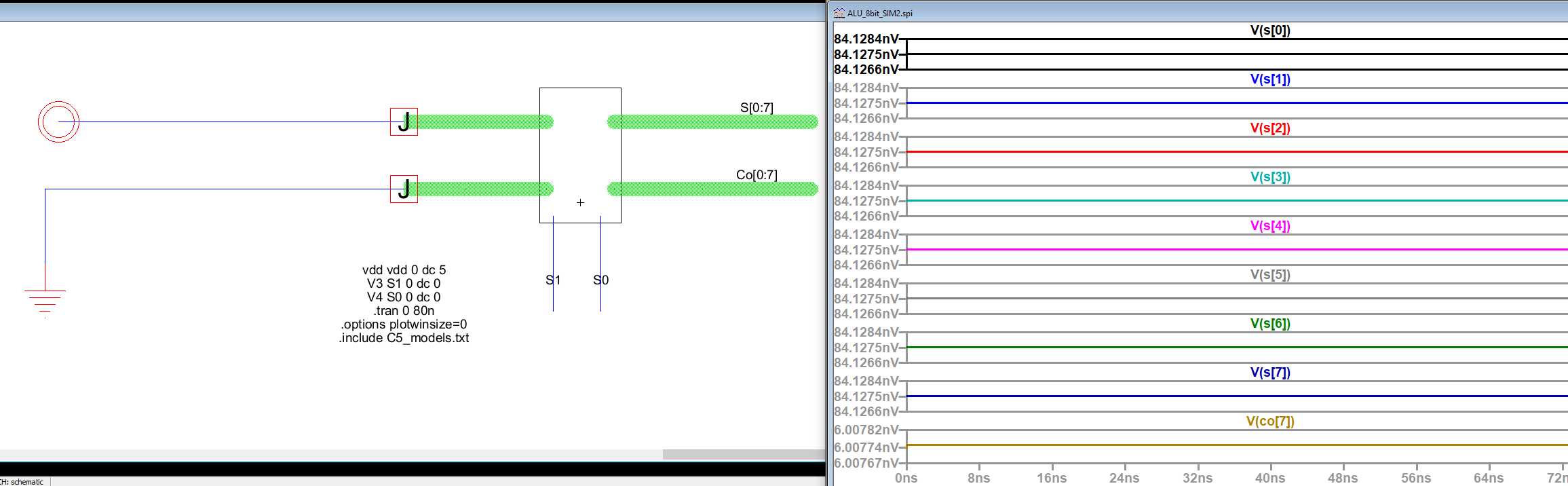Figure 4 - Simulating AND logic showing all 0s for output as expected.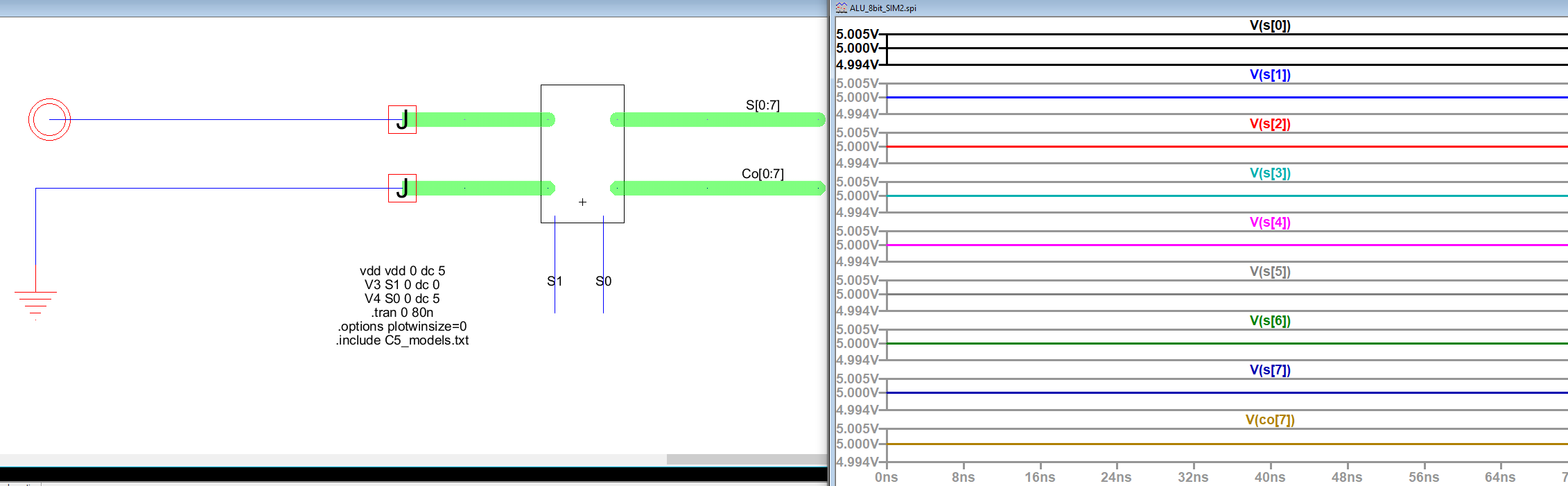Figure 5 - Simulating OR logic showing all 1s for output as expected.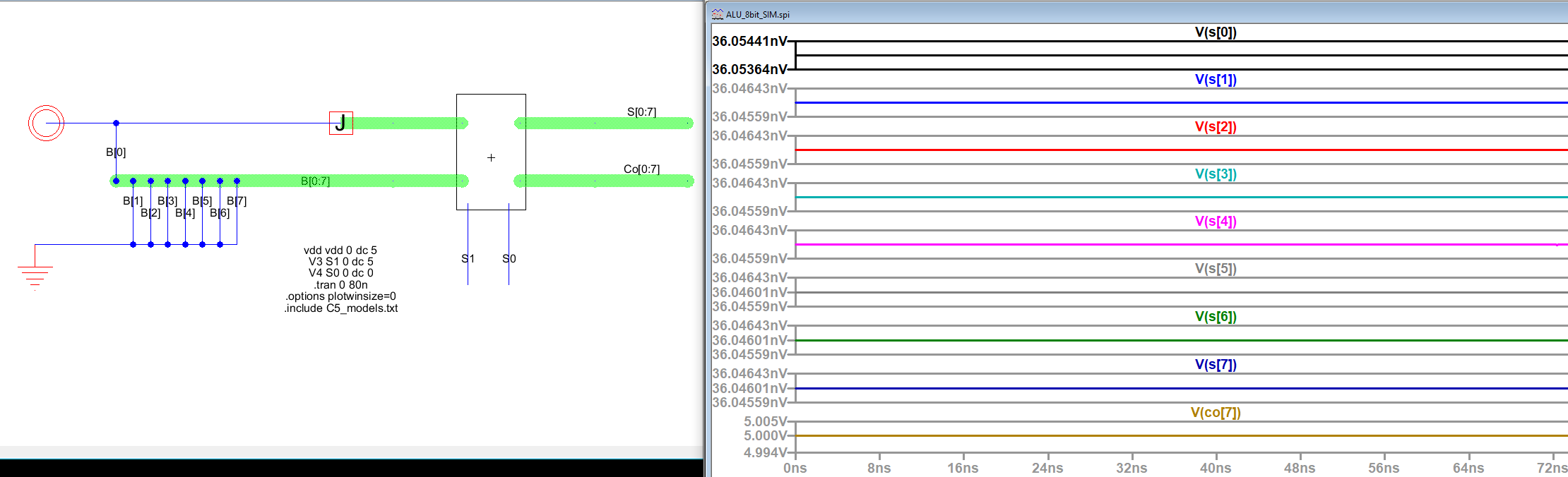Figure 6 - Simulating addition operation for 0xFF + 0x01 with result S=0x00 and Co=1 as expected.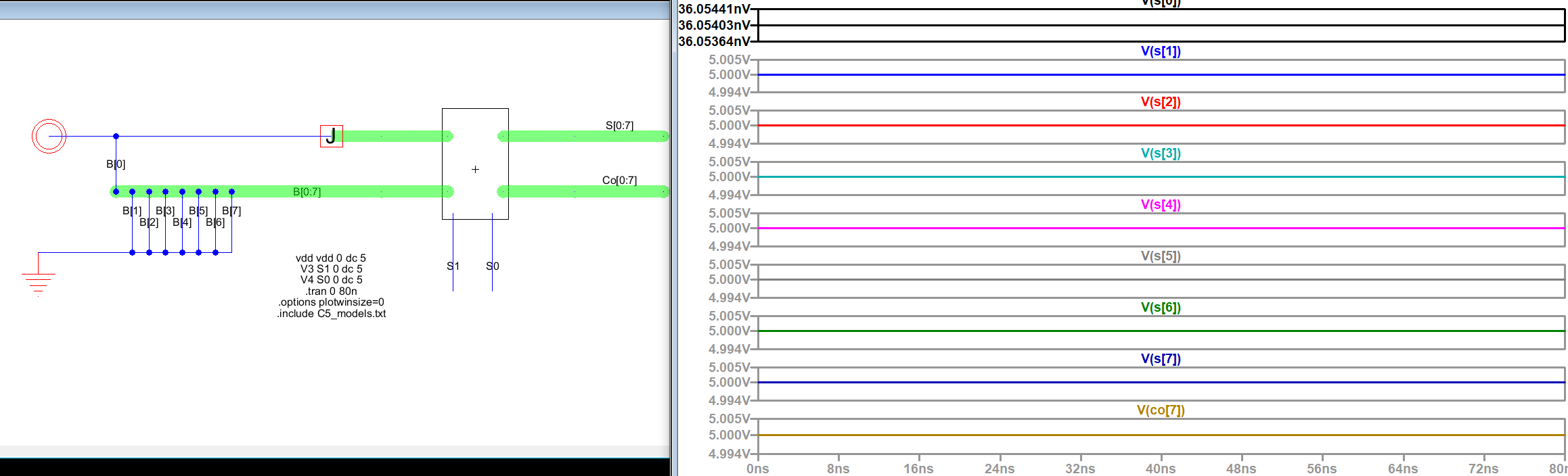Figure 7 - Simulating subraction with 0xFF-0x01w/ S=0xFE, Co=0x01 as expected.

Discussion

Unlike previous labs the 8-bit ALU logic was verified after the layout was completed since I forgot to verify it first. However, as Figures 4 through 7 show, the 8-bit ALU is operating as it should. The layout itself was was created without much issue, it was just time consuming to make sure all the wires was neat and spaced properly.